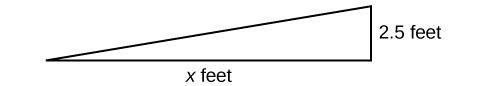## Section Exercises

1. What does it mean when we say that two lines are parallel?

2. What is the relationship between the slopes of perpendicular lines (assuming neither is horizontal nor vertical)?

3. How do we recognize when an equation, for example $y=4x+3$, will be a straight line (linear) when graphed?

4. What does it mean when we say that a linear equation is inconsistent?

5. When solving the following equation: $\frac{2}{x - 5}=\frac{4}{x+1}$, explain why we must exclude $x=5$ and $x=-1$ as possible solutions from the solution set.

For the following exercises, solve the equation for $x$.

6. $7x+2=3x - 9$

7. $4x - 3=5$

8. $3\left(x+2\right)-12=5\left(x+1\right)$

9. $12 - 5\left(x+3\right)=2x - 5$

10. $\frac{1}{2}-\frac{1}{3}x=\frac{4}{3}$

11. $\frac{x}{3}-\frac{3}{4}=\frac{2x+3}{12}$

12. $\frac{2}{3}x+\frac{1}{2}=\frac{31}{6}$

13. $3\left(2x - 1\right)+x=5x+3$

14. $\frac{2x}{3}-\frac{3}{4}=\frac{x}{6}+\frac{21}{4}$

15. $\frac{x+2}{4}-\frac{x - 1}{3}=2$

For the following exercises, solve each rational equation for $x$. State all x-values that are excluded from the solution set.

16. $\frac{3}{x}-\frac{1}{3}=\frac{1}{6}$

17. $2-\frac{3}{x+4}=\frac{x+2}{x+4}$

18. $\frac{3}{x - 2}=\frac{1}{x - 1}+\frac{7}{\left(x - 1\right)\left(x - 2\right)}$

19. $\frac{3x}{x - 1}+2=\frac{3}{x - 1}$

20. $\frac{5}{x+1}+\frac{1}{x - 3}=\frac{-6}{{x}^{2}-2x - 3}$

21. $\frac{1}{x}=\frac{1}{5}+\frac{3}{2x}$

For the following exercises, find the equation of the line using the point-slope formula. Write all the final equations using the slope-intercept form.

22. $\left(0,3\right)$ with a slope of $\frac{2}{3}$

23. $\left(1,2\right)$ with a slope of $\frac{-4}{5}$

24. x-intercept is 1, and $\left(-2,6\right)$

25. y-intercept is 2, and $\left(4,-1\right)$

26. $\left(-3,10\right)$ and $\left(5,-6\right)$

27. $\left(1,3\right)\text{ and }\left(5,5\right)$

28. parallel to $y=2x+5$ and passes through the point $\left(4,3\right)$

29. perpendicular to $\text{3}y=x - 4$ and passes through the point $\left(-2,1\right)$ .

For the following exercises, find the equation of the line using the given information.

30. $\left(-2,0\right)$ and $\left(-2,5\right)$

31. $\left(1,7\right)$ and $\left(3,7\right)$

32. The slope is undefined and it passes through the point $\left(2,3\right)$.

33. The slope equals zero and it passes through the point $\left(1,-4\right)$.

34. The slope is $\frac{3}{4}$ and it passes through the point $\left(1,4\right)$.

35. $\left(-1,3\right)$ and $\left(4,-5\right)$

For the following exercises, graph the pair of equations on the same axes, and state whether they are parallel, perpendicular, or neither.

36. $\begin{array}{l}\\ y=2x+7\hfill \\ y=\frac{-1}{2}x - 4\hfill \end{array}$

37. $\begin{array}{l}3x - 2y=5\hfill \\ 6y - 9x=6\hfill \end{array}$

38. $\begin{array}{l}y=\frac{3x+1}{4}\hfill \\ y=3x+2\hfill \end{array}$

39. $\begin{array}{l}x=4\\ y=-3\end{array}$

For the following exercises, find the slope of the line that passes through the given points.

40. $\left(5,4\right)$ and $\left(7,9\right)$

41. $\left(-3,2\right)$ and $\left(4,-7\right)$

42. $\left(-5,4\right)$ and $\left(2,4\right)$

43. $\left(-1,-2\right)$ and $\left(3,4\right)$

44. $\left(3,-2\right)$ and $\left(3,-2\right)$

For the following exercises, find the slope of the lines that pass through each pair of points and determine whether the lines are parallel or perpendicular.

45. $\begin{array}{l}\left(-1,3\right)\text{ and }\left(5,1\right)\\ \left(-2,3\right)\text{ and }\left(0,9\right)\end{array}$

46. $\begin{array}{l}\left(2,5\right)\text{ and }\left(5,9\right)\\ \left(-1,-1\right)\text{ and }\left(2,3\right)\end{array}$

For the following exercises, express the equations in slope intercept form (rounding each number to the thousandths place). Enter this into a graphing calculator as Y1, then adjust the ymin and ymax values for your window to include where the y-intercept occurs. State your ymin and ymax values.

47. $0.537x - 2.19y=100$

48. $4,500x - 200y=9,528$

49. $\frac{200 - 30y}{x}=70$

50. Starting with the point-slope formula $y-{y}_{1}=m\left(x-{x}_{1}\right)$, solve this expression for $x$ in terms of ${x}_{1},y,{y}_{1}$, and $m$.

51. Starting with the standard form of an equation $\text{A}x\text{ + B}y\text{ = C,}$ solve this expression for y in terms of $A,B,C$, and $x$. Then put the expression in slope-intercept form.

52. Use the above derived formula to put the following standard equation in slope intercept form: $7x - 5y=25$.

53. Given that the following coordinates are the vertices of a rectangle, prove that this truly is a rectangle by showing the slopes of the sides that meet are perpendicular.

$\left(-1,1\right),\left(2,0\right),\left(3,3\right)$, and $\left(0,4\right)$

54. Find the slopes of the diagonals in the previous exercise. Are they perpendicular?

55. The slope for a wheelchair ramp for a home has to be $\frac{1}{12}$. If the vertical distance from the ground to the door bottom is 2.5 ft, find the distance the ramp has to extend from the home in order to comply with the needed slope.56. If the profit equation for a small business selling $x$ number of item one and $y$ number of item two is $p=3x+4y$, find the $y$ value when $p=\453\text{ and }x=75$.

For the following exercises, use this scenario: The cost of renting a car is $45/wk plus$0.25/mi traveled during that week. An equation to represent the cost would be $y=45+.25x$, where $x$ is the number of miles traveled.

57. What is your cost if you travel 50 mi?

58. If your cost were $\63.75$, how many miles were you charged for traveling?

59. Suppose you have a maximum of \$100 to spend for the car rental. What would be the maximum number of miles you could travel?